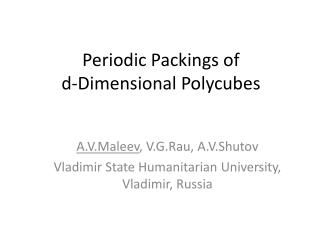# Periodic Packings of d-Dimensional Polycubes - PowerPoint PPT PresentationDownload PresentationPeriodic Packings of d-Dimensional Polycubes

Download Presentation## Periodic Packings of d-Dimensional Polycubes

- - - - - - - - - - - - - - - - - - - - - - - - - - - E N D - - - - - - - - - - - - - - - - - - - - - - - - - - -
##### Presentation Transcript

1. Periodic Packings of d-Dimensional Polycubes A.V.Maleev, V.G.Rau, A.V.Shutov Vladimir State Humanitarian University, Vladimir, Russia

2. Definitions • Polycube is a finite union of elementary cells of d-dimentional simple cubic lattice L with connected interior. • Centers of elementary cells from polycube are called polycube points. • The polycubes packing is called normal if all polycube points from the packing belong to L.

3. The polycube

4. Definitions • The polycubes packing is called periodic if its automorphism group contains some d-dimensional lattice Γ. • If the fundamental domain of Γ contains only single polycube we have a translation polycube packing. • If packing density k = 1 we have a polycube tiling.

5. Problem statement • Search of all possible variants of periodic polycubes packing for the given finite set of d-dimensional polycubes with the given packing density. • Search of all possible variants of d-dimensional periodic polycubes tiling with the given volume of translation fundamental domain.

6. Using Polycubes in Crystal Structure Prediction • Crystal structure prediction is the prediction of the equilibrium structure of a crystal on the basis of the known structure of molecules. • One of approaches of this problem is based on the close packing principle (A.I.Kitaigorodskii) for geometric models of molecules. • Approximation of molecules by polycubes reduces a crystal structure prediction problem to searching of periodic polycubespackings.

7. Approximation of molecule by polycube Geometric model Molecule Polycube Lattice points

8. Historical Background • Polyominoes are shapes made by connecting certain numbers of equal-sized squares, each joined together with at least one other square along an edge (Golomb, 1953). • Translation criterion and Conway’s criterion of existence of a periodic tiling of the plane for given polyomino (Conway). • Using this criterions Rhoads (2005) enumerates and classifies the tilings for small polyominoes (n≤9).

9. Packing Space A packing space is the pair (L;w), where L is a lattice, and w is a function w : L → {0; 1;…n-1} such that all sets w-1(i), i = 0; 1;…; n-1 are equivalent by translation to some sublattice Γ in lattice L. For any lattice point xw(x) is called the weight of this point. The number n is called the order of packing space. It is obvious that n=[L:Γ].

10. Packing Space

11. Packing Space

12. Packing Space

13. Packing Space

14. Theorem 1

15. Algorithm 1

16. Algorithm 1

17. Complexity of Algorithm 1

18. Algorithm 2

19. Algorithm 2

20. Algorithm 2

21. Packing Code

22. Packing Code 3 2 1 0

23. Packing Code 3 2 0 2 1 3 1

24. 0 1 2 3 4 5 6 3 2 0 2 1 3 1 Packing Code

25. The numbers tZ(n) of periodic tilings of the plane on Z polyominoes with volume of the fundamental domain equals to n

26. Publications • Maleev A.V., Rau V.G., Potekhin K.A., Parkhomov L.G., Rau T.F., Stepanov S.V., StruchkovYu.T. Discrete modeling method in molecular crystals. // Dokl. Akad. Nauk SSSR, 1990, 315, 1382-1385. • Potekhin K.A., Maleev A.V. Molecular cells in organic crystals. // Dokl. Akad. Nauk SSSR, 1991, 318, 1170-1173. • Maleev A.V. n-Dimensional pacing space. // Crystallography Reports, 1995, 40. • Maleev A.V., Lysov A.E., Potekhin K.A. Symmetry n -Dimensional pacing space. // Crystallography Reports 1998, 43, 721-727. • Maleev A.V. An Algorithm and Program of Exhaustive Search for Possible Tiling of a Plane with Polyominoes. // Crystallography Reports, 2001, 46, 154-156. • Maleev A.V. Generation of Molecular Bravais Structures by the Method of Discrete Modeling of Packings.Crystallography Reports, 46, 2001, 13–18. • Maleev A.V. Generation of the Structures of Molecular Crystals with Two Molecules Related by the Center of Inversion in a Primitive Unit Cell. // Crystallography Reports, 47, 2002, 731–735. • Maleev A.V. Generation of Structures of Molecular Crystals with Two Molecules Related by a Twofold Axis or a Plane of Symmetry in a Primitive Unit Cell. // Crystallography Reports, 2006, 51, 559–563.

27. Thank you for attention!